• leastsq
2022-03-04 22:23:06

### 1. 问题:

已知三维空间的一些点集，求拟合出来的平面； 也就是求出所有点到平面的距离最短的平面参数；

### 2. 最优化的问题：

依旧是说，使用最小二乘法，得到平面的参数；

### 3. 函数说明：

直接使用 python scipy optimize： leastsq 方法的用法
leastaq 官方函数说明
简化说明：

• scipy.optimize.leastsq(func, x0, args=(), Dfun=None, full_output=0, col_deriv=0, ftol=1.49012e-08, xtol=1.49012e-08, gtol=0.0, maxfev=0, epsfcn=None, factor=100, diag=None)
• func 表示估计函数
• x0 表示初始的估计参数
• args 表示函数的参数

### 4. 代码：

import numpy as np
from scipy.optimize import leastsq

def fit_func(p, x, y):
""" 数据拟合函数 """
a, b, c = p
return a * x + b * y + c

def residuals(p, x, y, z):
""" 误差函数 """
return z - fit_func(p, x, y)

def estimate_plane_with_leastsq(pts):
""" 根据最小二乘拟合出平面参数 """
p0 = [1, 0, 1]
np_pts = np.array(pts)
plsq = leastsq(residuals, p0, args=(np_pts[:, 0], np_pts[:, 1], np_pts[:, 2]))
return plsq

def get_proper_plane_params(p, pts):
""" 根据拟合的平面的参数，得到实际显示的最佳的平面 """
assert isinstance(pts, list), r'输入的数据类型必须依赖 list'
np_pts = np.array(pts)

np_pts_mean = np.mean(np_pts, axis=0)
np_pts_min = np.min(np_pts, axis=0)
np_pts_max = np.max(np_pts, axis=0)

plane_center_z = fit_func(p, np_pts_mean, np_pts_mean)
plane_center = [np_pts_mean, np_pts_mean, plane_center_z]

plane_origin_z = fit_func(p, np_pts_min, np_pts_min)
plane_origin = [np_pts_min, np_pts_min, plane_origin_z]

if np.linalg.norm(p) < 1e-10:
print(r'plsq 的 norm 值为 0 {}'.format(p))
plane_normal = p / np.linalg.norm(p)

plane_pt_1 = [np_pts_max, np_pts_min, fit_func(p, np_pts_max, np_pts_min)]
plane_pt_2 = [np_pts_min, np_pts_max, fit_func(p, np_pts_min, np_pts_max)]
return plane_center, plane_normal, plane_origin, plane_pt_1, plane_pt_2

if __name__ == '__main__':

pts = [
[0, 0, 128],
[256, 0, 128],
[256, 256, 128],
[0, 256, 128],
]

p = estimate_plane_with_leastsq(pts)
center, normal, origin, plane_x_pt, plane_y_pt = get_proper_plane_params(p, pts)
print(r'estimate plane center: {}, normal: {}, origin: {}, plane_x_pt: {}, plane_y_pt: {}'.format(
center, normal, origin, plane_x_pt, plane_y_pt))



### 5. 结果：

estimate plane
center: [128.0, 128.0, 128.0],
normal: [-1.70384540e-14  1.41639579e-18  1.00000000e+00],
origin: [0, 0, 128.00000000027913],
plane_x_pt: [256, 0, 127.99999999972081],
plane_y_pt: [0, 256, 128.0000000002792]


输出结果与预期的结果是一致的；python 最小二乘法 scipy
更多相关内容
• 可以看到这一个问题实际上还是一个优化问题，也可以用之前拟合函数的leastsq求解。下面用这两个方法进行对比： 代码： from scipy.optimize import fsolve,leastsq from math import sin,cos def f(x): x0 = float...
• leastsq作用：最小化一组方程的平方和。 参数设置： func 误差函数 x0 初始化的参数 args 其他的额外参数 举个例子就清楚了 首先创建样本点 import numpy as np import scipy as sp from scipy.optimize import ...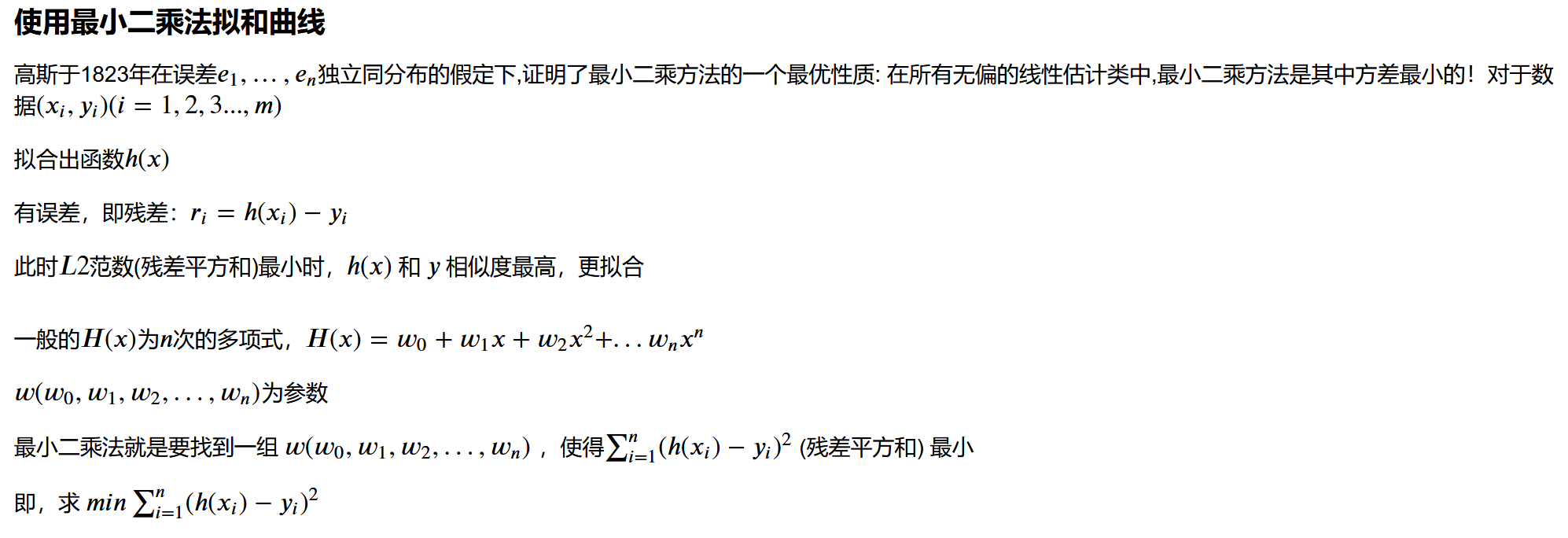leastsq作用：最小化一组方程的平方和。
参数设置：
func 误差函数
x0 初始化的参数
args 其他的额外参数

举个例子就清楚了
首先创建样本点

import numpy as np
import scipy as sp
from scipy.optimize import leastsq
import matplotlib.pyplot as plt
plt.rcParams['font.sans-serif']=['SimHei']
plt.rcParams['axes.unicode_minus']=False
x=[1,2,3,4]
y=[2,3,4,5]


拟合直线

def y_pre(p,x):
f=np.poly1d(p)
return f(x)


其中的np.polyld

f=np.poly1d([1,2,3])
# x^2+2x+3
f(1)
"""
6
"""


误差函数

def error(p,x,y):
return y-y_pre(p,x)


接下就简单了

p=[1,2]    # 值随便写
# y=w1*x+w2
res=leastsq(error,p,args=(x,y))
w1,w2=res   # res中就是wi的参数列表
"""
到这w1和w2就已经求出来了，下面是画图看一下
"""
x_=np.linspace(1,10,100)   # 等差数列，
y_p=w1*x_+w2               # 求出的拟合曲线
plt.scatter(x,y)           # 样本点
plt.plot(x_,y_p)           # 画拟合曲线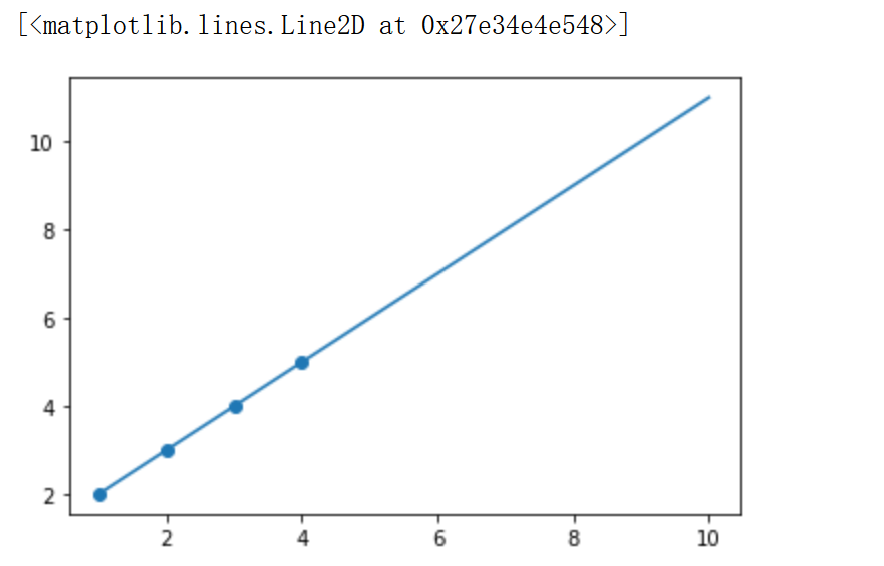可以直接封装成函数

x=np.linspace(0,2,10)
y=np.sin(np.pi*x)
# 原始的样本
y_=[y + np.random.normal(0,0.1) for y in y]     # np.random.normal(loc,scale,size):正态分布的均值,正态分布的标准差,形状

# np.random.randn()   # 标准正态分布是以0为均数、以1为标准差的正态分布，记为N（0，1）

def fit(M=1):
p=np.random.rand(M+1)   # 返回一个或一组服从“0~1”均匀分布的随机样本值。随机样本取值范围是[0,1)
res=leastsq(error,p,args=(x,y))  # wi 的值
x_point=np.linspace(0,2,100)  # 增加数据量为了画出的图平滑
y_point=np.sin(np.pi*x_point) # 增加数据量为了画出的图平滑
plt.plot(x_point,y_point,'r',label='原始')
plt.plot(x_point,y_pre(res,x_point),'b',label='拟合')
plt.scatter(x,y_)
plt.legend()
fit(3)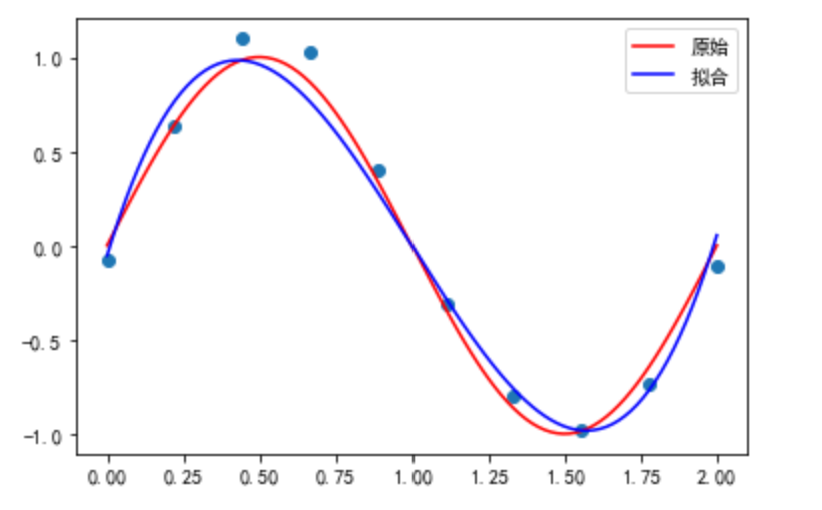你也可以输出一下中间的结果

x=np.linspace(0,2,10)
y=np.sin(np.pi*x)
# 原始的样本
y_=[y + np.random.normal(0,0.1) for y in y]     # np.random.normal(loc,scale,size):正态分布的均值,正态分布的标准差,形状

# np.random.randn()   # 标准正态分布是以0为均数、以1为标准差的正态分布，记为N（0，1）

def fit(M=1):
p=np.random.rand(M+1)   # 返回一个或一组服从“0~1”均匀分布的随机样本值。随机样本取值范围是[0,1)
res=leastsq(error,p,args=(x,y))  # wi 的值
x_point=np.linspace(0,2,100)
y_point=np.sin(np.pi*x_point)
plt.plot(x_point,y_point,'r',label='原始')
plt.plot(x_point,y_pre(res,x_point),'b',label='拟合')
print(res)
plt.scatter(x,y_)
plt.legend()
fit(3)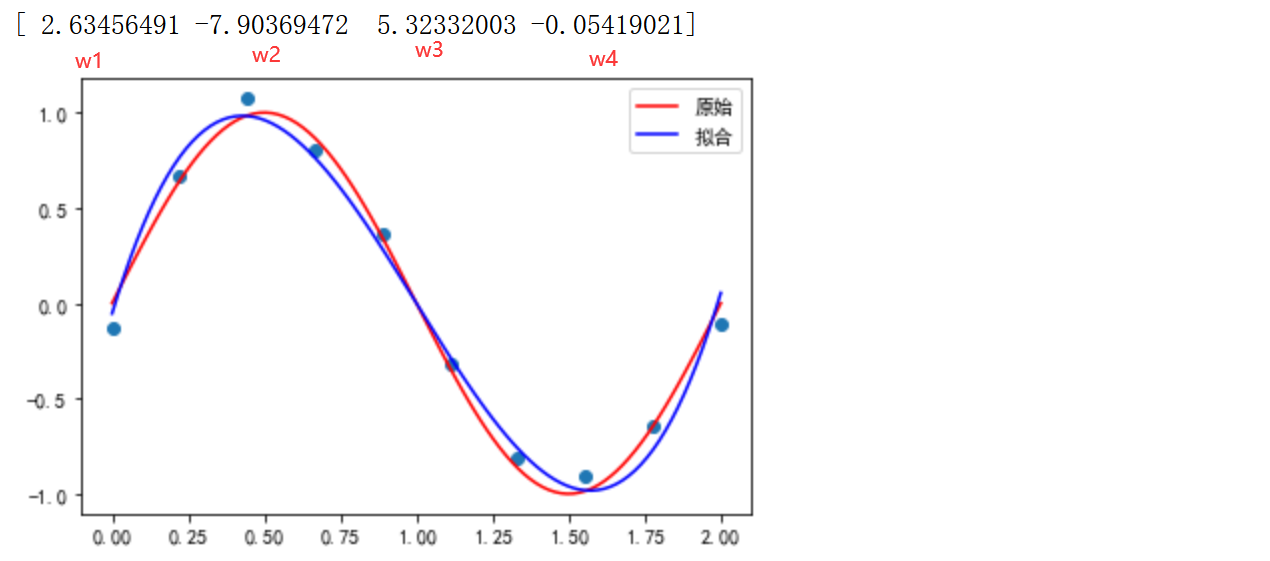拟合的直线就是
y = w 1 ∗ x 3 + w 2 ∗ x 2 + w 3 ∗ x 1 + w 4 ∗ x 0 y=w_1*x^3+w_2*x^2+w3*x^1+w_4*x^0

展开全文python 机器学习
• python中scipy.optimize.leastsq（最小二乘拟合）用法    《Python程序设计与科学计算》中SciPy.leastsq（最小二乘拟合）的一些笔记。    假设有一组实验数据(xi,yi)，已知它们之间的函数关系为y=f(x)，通过这些...

## python中scipy.optimize.leastsq（最小二乘拟合）用法

《Python程序设计与科学计算》中SciPy.leastsq（最小二乘拟合）的一些笔记。
假设有一组实验数据(xi,yi)，已知它们之间的函数关系为y=f(x)，通过这些信息，需要确定函数中的一些参数项。例如，如果f是一个线性函数f(x)=kx+b，那么参数kb就是需要确定的值，得到如下公式中的S函数最小：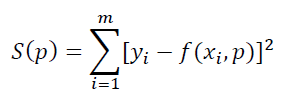这种算法被称为最小二乘拟合（Least Square Fitting）。
SciPy子函数库optimize已经提供了实现最小二乘拟合算法的函数leastsq。下面的例子使用leastsq，实现最小二乘拟合。
定义数据拟合所用函数：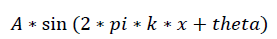import numpy as np
from scipy import linalg
from scipy.optimize import leastsq

def func(x, p):
A, k, theta = p
return A * np.sin(2 * np.pi * k * x + theta)


下面代码是定义计算误差函数，计算实验数据想x、y和拟合函数之间的差，参数p为拟合需要找到的系数，同时添加噪声数据。

def residuals(p, y, x):
return y - func(x, p)

x = np.linspace(0, -2 * np.pi, 100)
A, k, theta = 10, 0.34, np.pi / 6                     # 真实数据的函数参数
y0 = func(x, [A, k, theta])                           # 真实数据
y1 = y0 + 2 * np.random.randn(len(x))                 # 加入噪声之后的实验数据
p0 = [7, 0.2, 0]                                      # 第一次猜测的函数拟合参数


调用leastsq拟合，residuals为计算误差函数，p0为拟合参数初始值，args为需要拟合的实验数据。

plsq = leastsq(residuals, p0, args=(y1, x))
print("真实参数:", [A, k, theta])
print("拟合参数:", plsq)


输出：
真实参数: [10, 0.34, 0.5235987755982988]
拟合参数: [10.56295783 0.33945091 0.46569609]
从结果可以看出，最小二乘拟合的结果与真实值之间的误差在可控范围之内，模型具有较好的效果。下面通过绘制图像来观察数据拟合效果，如图1所示。

import matplotlib.pyplot as plt
import pylab as pl

plt.rcParams['font.sans-serif'] = ['SimHei']          # 用来正常显示中文标签
plt.rcParams['axes.unicode_minus'] = False            # 用来正常显示负号
pl.plot(x, y0, marker='+', label=u"真实数据")
pl.plot(x, y1, marker='^', label=u"带噪声的实验数据")
pl.plot(x, func(x, plsq), label=u"拟合数据")
pl.legend()
pl.show()完整代码如下：

import numpy as np
from scipy import linalg
from scipy.optimize import leastsq

def func(x, p):
A, k, theta = p
return A * np.sin(2 * np.pi * k * x + theta)

def residuals(p, y, x):
return y - func(x, p)

x = np.linspace(0, -2 * np.pi, 100)
A, k, theta = 10, 0.34, np.pi / 6                     # 真实数据的函数参数
y0 = func(x, [A, k, theta])                           # 真实数据
y1 = y0 + 2 * np.random.randn(len(x))                 # 加入噪声之后的实验数据
p0 = [7, 0.2, 0]                                      # 第一次猜测的函数拟合参数

plsq = leastsq(residuals, p0, args=(y1, x))
print("真实参数:", [A, k, theta])
print("拟合参数:", plsq)

import matplotlib.pyplot as plt
import pylab as pl

plt.rcParams['font.sans-serif'] = ['SimHei']          # 用来正常显示中文标签
plt.rcParams['axes.unicode_minus'] = False            # 用来正常显示负号
pl.plot(x, y0, marker='+', label=u"真实数据")
pl.plot(x, y1, marker='^', label=u"带噪声的实验数据")
pl.plot(x, func(x, plsq), label=u"拟合数据")
pl.legend()
pl.show()


关于leastsq()的说明：
scipy.optimize.leastsq(func, x0, args=(), Dfun=None, full_output=0, col_deriv=0, ftol=1.49012e-08, xtol=1.49012e-08, gtol=0.0, maxfev=0, epsfcn=None, factor=100, diag=None)

参数：
（1）func：callable
应该至少接受一个(可能为N个向量)长度的参数，并返回M个浮点数。它不能返回NaN，否则拟合可能会失败。
func 是我们自己定义的一个计算误差的函数。

（2）x0：ndarray
最小化的起始估算。
x0 是计算的初始参数值。

（3）args：tuple, 可选参数
函数的所有其他参数都放在此元组中。
args 是指定func的其他参数。

一般我们只要指定前三个参数就可以了。

（4）Dfun：callable, 可选参数
一种计算函数的雅可比行列的函数或方法，其中行之间具有导数。如果为None，则将估算雅可比行列式。

（5）full_output：bool, 可选参数
非零可返回所有可选输出。

（6）col_deriv：bool, 可选参数
非零，以指定Jacobian函数在列下计算导数(速度更快，因为没有转置操作)。

（7）ftol：float, 可选参数
期望的相对误差平方和。

（8）xtol：float, 可选参数
近似解中需要的相对误差。

（9）gtol：float, 可选参数
函数向量和雅可比行列之间需要正交。

（10）maxfev：int, 可选参数
该函数的最大调用次数。如果提供了Dfun，则默认maxfev为100 *(N + 1)，其中N是x0中的元素数，否则默认maxfev为200 *(N + 1)。

（11）epsfcn：float, 可选参数
用于确定合适的步长以进行雅可比行进的正向差分近似的变量(对于Dfun = None)。通常，实际步长为sqrt(epsfcn)* x如果epsfcn小于机器精度，则假定相对误差约为机器精度。

（12）factor：float, 可选参数
决定初始步骤界限的参数(factor * || diag * x||)。应该间隔(0.1, 100)。

（13）diag：sequence, 可选参数
N个正条目，作为变量的比例因子。

返回值：
（1）x：ndarray
解决方案(或调用失败的最后一次迭代的结果)。

（2）cov_x：ndarray
黑森州的逆。 fjac和ipvt用于构造粗麻布的估计值。无值表示奇异矩阵，这意味着参数x的曲率在数值上是平坦的。要获得参数x的协方差矩阵，必须将cov_x乘以残差的方差-参见curve_fit。

（3）infodict：字典
带有键的可选输出字典：

（4）nfev
函数调用次数

（5）fvec
在输出处评估的函数

（6）fjac
最终近似雅可比矩阵的QR因式分解的R矩阵的排列，按列存储。与ipvt一起，可以估算出估计值的协方差。

（7）ipvt
长度为N的整数数组，它定义一个置换矩阵p，以使fjac * p = q * r，其中r是上三角形，其对角线元素的幅度没有增加。 p的第j列是单位矩阵的ipvt(j)列。

（8）qtf
向量(transpose(q)* fvec)。

（9）mesg：力量
字符串消息，提供有关失败原因的信息。

（10）ier：整型
整数标志。如果等于1、2、3或4，则找到解。否则，找不到解决方案。无论哪种情况，可选输出变量‘mesg’都会提供更多信息。

关于leastsq()的说明转载自：https://vimsky.com/examples/usage/python-scipy.optimize.leastsq.html

展开全文python
• 拟合方法——leastsq 1. 概念: scipy官网对该方法介绍是: 最小化一组方程的平方和 x=arg⁡min⁡y(∑((func(y))2，axis=0))x =\arg \min\limits_{y}(\sum((func(y))^2，axis=0))x=argymin​(∑((func(y))2，axis=0)) ...

## 拟合方法——leastsq

### 1. 概念:

scipy官网对该方法介绍是: 最小化一组方程的平方和
x = arg ⁡ min ⁡ y ( ∑ ( ( f u n c ( y ) ) 2 ， a x i s = 0 ) ) x =\arg \min\limits_{y}(\sum((func(y))^2，axis=0))
简单介绍一下leastsq的参数:
scipy.optimize.leastsq（func，x0，args = （），Dfun = None，full_output = 0，col_deriv = 0，ftol = 1.49012e-08，xtol = 1.49012e-08，gtol = 0.0，maxfev = 0，epsfcn = None，factor = 100，diag = None）

# 参数:
func: 所求平方和最小值的函数，一般都用于拟合时传入残差函数，当然也有其他用途，比如求函数的最小等等
x0: ndarray  一般为参数的初始值
args: 元组，可选，可以传入(x, y)其中x,y都是数组，作为求解传入的参数

# 返回值
leastsq的返回值是一个元组，里面包含了很多信息，如果是用作拟合的话，返回值的索引值为0的元素即为我们所求函数参数


有兴趣的读者可以自己看看官网介绍: leastsq官网

### 2. 使用:

##### 1. 求最小值

比如求 2 ( x − 3 ) 2 + 1 2(x-3)^2+1 的最小值:

from scipy.optimize import leastsq

def func(x):
return 2*(x-3)**2+1
print(leastsq(func, 0))   # 0为x的初始值，初始值可以由自己定,改成任意一个随机值都可以


结果为:

(array([2.99999999]), 1)


x = 2.99999999 ≈ 3 x=2.99999999\approx3 时，函数最小值为1

##### 2. 拟合

为了和上一篇文章数学建模方法—【04】拟合方法之np.polyfit、np.poly1d形成一个比较，我们这里也进行1-5阶的多项式拟合。

1. 定义要拟合的函数关系式 (因为我们这里函数关系式是多项式，所以就用np.ploy1d获取了多项式了)
from scipy.optimize import leastsq
# 拟合数据集
x = [4, 8, 10, 12, 25, 32, 43, 58, 63, 69, 79]
y = [20, 33, 50, 56, 42, 31, 33, 46, 65, 75, 78]
x_arr = np.array(x)
y_arr = np.array(y)

def fitting_func(p, x):
"""
获得拟合的目标数据数组
:param p: array[int] 多项式各项从高到低的项的系数数组
:param x: array[int] 自变量数组
:return: array[int] 拟合得到的结果数组
"""
f = np.poly1d(p)    # 获得拟合后得到的多项式
return f(x)         # 将自变量数组带入多项式计算得到拟合所得的目标数组

如果想要拟合的是其他函数，那只需要将 f = np.poly1d(p)改为你想要拟合的函数关系式即可。
2. 定义残差计算函数
def error_func(p, x, y):
"""
计算残差
:param p: array[int] 多项式各项从高到低的项的系数数组
:param x: array[int] 自变量数组
:param y: array[int] 原始目标数组(因变量)
:return: 拟合得到的结果和原始目标的差值
"""
err = fitting_func(p, x) - y
return err

残差有很多计算方法，这里因为leastsq方法本身就会将我们的残差函数平方，所以这里我们直接相减，在经过leastsq方法的平方后就变为了最小二乘法了。
3. 获取参数
def n_poly(n, x, y):
"""
n 次多项式拟合函数
:param n: 多项式的项数(包括常数项)，比如n=3的话最高次项为2次项
:return: 最终得到的系数数组
"""
p_init = np.random.randn(n)   # 生成 n个随机数，作为各项系数的初始值，用于迭代修正
parameters = leastsq(error_func, p_init, args=(np.array(x), np.array(y)))    # 三个参数：误差函数、函数参数列表、数据点
return parameters	# leastsq返回的是一个元组，元组的第一个元素时多项式系数数组[wn、...、w2、w1、w0]

4. 函数定义好后，就可以进行拟合了(这里计算拟合优度的方法goodness_of_fit在我前面的文章有: 数学建模方法—【03】拟合优度的计算(python计算))
一阶
para_2 = n_poly(2, x_arr, y_arr)  # 一阶
print("系数为:", para_2)
print("多项式为:", np.poly1d(para_2))
print("拟合值为:", fitting_func(para_2, x_arr))
print("拟合优度为:", goodness_of_fit(fitting_func(para_2, x_arr), y_arr))

二、三、四、五阶:
para_3 = n_poly(3, x_arr, y_arr)  # 二阶
para_4 = n_poly(4, x_arr, y_arr)  # 三阶
para_5 = n_poly(5, x_arr, y_arr)  # 四阶
para_6 = n_poly(6, x_arr, y_arr)  # 五阶

这里解释一下为什么一阶传入n=2，二阶传入n=3…。因为这里的n表示的不是多项式的阶数，而是表示多项式的项数，比如二阶的多项式有 x 2 、 x x^2、x 还有常数项三项，所以传入的n=3。
5. 得到拟合的数据后就可以作图了
figure = plt.figure(figsize=(8,6))
plt.plot(x_arr, fitting_func(para_2, x_arr), color="#72CD28", label='一阶拟合曲线')
plt.plot(x_arr, fitting_func(para_3, x_arr), color="#EBBD43", label='二阶拟合曲线')
plt.plot(x_arr, fitting_func(para_4, x_arr), color="#50BFFB", label='三阶拟合曲线')
plt.plot(x_arr, fitting_func(para_5, x_arr), color="gold", label='四阶拟合曲线')
plt.plot(x_arr, fitting_func(para_6, x_arr), color="#13EAC9", label='五阶拟合曲线')
plt.scatter(x, y, color='#50BFFB', marker="p", label='原始数据')

plt.title("leastsq拟合", fontsize=13)
plt.xlabel('x', fontsize=13)
plt.ylabel('y', fontsize=13)
plt.legend(loc=4, fontsize=11)    # 指定legend的位置右下角
plt.rcParams['font.sans-serif'] = ['SimHei']  # 用来正常显示中文标签
plt.rcParams['axes.unicode_minus'] = False  # 用来正常显示负号
plt.show()

这里我只输出了一阶的拟合结果，结果为:
系数为: [ 0.5000123  29.77227653]
多项式为:
0.5 x + 29.77
拟合值为: [31.77232574 33.77237495 34.77239955 35.77242415 42.27258408 45.77267019		 51.27280552 58.77299004 61.27305155 64.27312537 69.27324839]
拟合优度为: 0.5207874477394239

结果图为:全部代码为:

from scipy.optimize import leastsq
# 拟合数据集
x = [4, 8, 10, 12, 25, 32, 43, 58, 63, 69, 79]
y = [20, 33, 50, 56, 42, 31, 33, 46, 65, 75, 78]
x_arr = np.array(x)
y_arr = np.array(y)
def fitting_func(p, x):
"""
获得拟合的目标数据数组
:param p: array[int] 多项式各项从高到低的项的系数数组
:param x: array[int] 自变量数组
:return: array[int] 拟合得到的结果数组
"""
f = np.poly1d(p)    # 获得拟合后得到的多项式
return f(x)         # 将自变量数组带入多项式计算得到拟合所得的目标数组

def error_func(p, x, y):
"""
计算残差
:param p: array[int] 多项式各项从高到低的项的系数数组
:param x: array[int] 自变量数组
:param y: array[int] 原始目标数组(因变量)
:return: 拟合得到的结果和原始目标的差值
"""
err = fitting_func(p, x) - y
return err

def n_poly(n, x, y):
"""
n 次多项式拟合函数
:param n: 多项式的项数(包括常数项)，比如n=3的话最高次项为2次项
:return: 最终得到的系数数组
"""
p_init = np.random.randn(n)   # 生成 n个随机数，作为各项系数的初始值，用于迭代修正
parameters = leastsq(error_func, p_init, args=(np.array(x), np.array(y)))    # 三个参数：误差函数、函数参数列表、数据点
return parameters	# leastsq返回的是一个元组，元组的第一个元素时多项式系数数组[wn、...、w2、w1、w0]

para_2 = n_poly(2, x_arr, y_arr)  # 一阶
print("系数为:", para_2)
print("多项式为:", np.poly1d(para_2))
print("拟合值为:", fitting_func(para_2, x_arr))
print("拟合优度为:", goodness_of_fit(fitting_func(para_2, x_arr), y_arr))
para_3 = n_poly(3, x_arr, y_arr)  # 二阶
para_4 = n_poly(4, x_arr, y_arr)  # 三阶
para_5 = n_poly(5, x_arr, y_arr)  # 四阶
para_6 = n_poly(6, x_arr, y_arr)  # 五阶
figure2 = plt.figure(figsize=(8,6))
plt.plot(x_arr, fitting_func(para_2, x_arr), color="#72CD28", label='一阶拟合曲线')
plt.plot(x_arr, fitting_func(para_3, x_arr), color="#EBBD43", label='二阶拟合曲线')
plt.plot(x_arr, fitting_func(para_4, x_arr), color="#50BFFB", label='三阶拟合曲线')
plt.plot(x_arr, fitting_func(para_5, x_arr), color="gold", label='四阶拟合曲线')
plt.plot(x_arr, fitting_func(para_6, x_arr), color="#13EAC9", label='五阶拟合曲线')
plt.scatter(x_arr, y_arr, color='#50BFFB', marker="p", label='原始数据')

plt.title("leastsq拟合", fontsize=13)
plt.xlabel('x', fontsize=13)
plt.ylabel('y', fontsize=13)
plt.legend(loc=4, fontsize=11)    # 指定legend的位置右下角
plt.rcParams['font.sans-serif'] = ['SimHei']  # 用来正常显示中文标签
plt.rcParams['axes.unicode_minus'] = False  # 用来正常显示负号
plt.show()

展开全文python
• 于4/6/2016更新在大多数情况下，在拟合参数中获得正确的错误可能是微妙的。让我们考虑一下拟合一个你有一组数据点(x_i，y_i，yerr_i)的函数y = f(x)，其中i是在每个数据...optimize.leastsq方法将返回分数协方差矩阵...
• https://blog.csdn.net/sinat_34328764/article/details/85473276最近接触到了Scipy中optimize模块的一些函数，optimize模块中提供了很多数值...在optimize模块中，使用leastsq()函数可以很快速地使用最小二乘法对数...
• 而在本篇博客中，我们介绍一个scipy库中的函数，叫leastsq，它可以省去中间那些具体的求解步骤，只需要输入一系列样本点，给出待求函数的基本形状（如我刚才所说，二元二次函数就是一种形状——f(x,y)=w0x ...最小二乘法
• 只需要输入一系列样本点，给出待求函数的基本形状（如二元二次函数就是一种形状 f(x,y)=w0x^2 + w1y^2 + w2xy + w3x + w4y + w5 ...则使用leastsq函数求解其拟合直线的代码如下： ###最小二乘法试验### import numpy...python
• 【Scipy教程】优化和拟合库scipy.optimize.leastsq()   优化是找到最小值或等式的数值解的问题。scipy.optimization子模块提供了函数最小值(标量或多维)、曲线拟合和寻找等式的根的有用算法。 最小二乘拟合 scipy....
• Python 闲谈二聊聊最小二乘法以及 leastsq 函数 最小二乘法概述 自从开始做毕设以来发现自己无时无刻不在接触最小二乘 法从求解线性透视图中的消失点 m 元 n 次函数的拟合 包括后来学到的神经网络其思想归根结底全都...
• 1、leastsq()与curve_fit() 1.1 leastsq()   Python中的leastsq()、curve_fit()拟合函数在scipy.optimize模块中，使用时需要导入。   leastsq()使用最小二乘方法，函数具体形式可以参考官方文档leastsq() 函数，...python
• leastsq apply for uwb locate self py just dont get it
• python用fsolve、leastsq对非线性方程组求解总结背景：实现用python的optimize库的fsolve对非线性方程组进行求解。可以看到这一个问题实际上还是一个优化问题，也可以用之前拟合函数的leastsq求解。下面用这两个方法...
• scipy.optimize.leastsq官方文档 之前自己用最小二乘进行过球面、柱面和平面拟合，球面和平面效果都很好，但是柱面效果一直不好（对初值十分敏感，迭代过程中经常出现奇异矩阵，也有可能是自己写的算法没有考虑数值...
• leastsq()函数位于Scipy的optimize模块中，利用leastsq()函数可以对数据进行拟合。 leastsq()函数的参数： leastsq(func,x0,args=()…)，其中常用的是前三个参数。 参数的介绍： p_init = np.random.rand(M + 1) # M...机器学习
• python 拟合 leastsq 最小二乘python 拟合 leastsq 最小二乘 python 拟合 leastsq 最小二乘 scipy库提供了函数leastsq函数用于非常规函数的参数拟合 代码实现如下： import numpy as np from scipy.optimize import ...python
• from scipy.optimize import leastsq import matplotlib.pyplot as plt def func(x,p): #数据拟合所用函数A*sin(2*pi*k*x+theta) A,k,theta=p return A*np.sin(2*np.pi*k*x+theta) def residuals(p,x,y): #实验...python
• scipy.optimize.leastsq leastsq(func, x0, args=(), Dfun=None, full_output=0, col_deriv=0, ftol=1.49012e-08, xtol=1.49012e-08, gtol=0.0, maxfev=0, epsfcn=0.0, factor=100, diag=None, warning=True) 只...
• optimize.leastsq能否将待拟合的参数限定在某个范围，比如μa,mμs都大于0。 代码： import numpy as np from scipy import optimize def farrell(r,p): #数据所拟合的函数，Farrell漫反射反演模型 ...python
• 23 s="Test the number of iteration" #试验最小二乘法函数leastsq得调用几次error函数才能找到使得均方误差之和最小的a~c 24 Para=leastsq(error,p0,args=(Xi,Yi,s)) #把error函数中除了p以外的参数打包到args中 25...
• 函数原型是 leastsq(func, x0, args=(), Dfun=None, full_output=0, col_deriv=0, ftol=1.49012e-08, xtol=1.49012e-08, gtol=0.0, maxfev=0, epsfcn=0.0, factor=100, diag=None, warning=True) leastsq(func, x0, ...python scipy 机器学习
• 使用scripy.optimize的leastsq求解拟合方程 import numpy as np from scipy . optimize import leastsq """target Func""" def real_func ( x ) : return np . sin ( 2 * np . pi * x ) "...机器学习
• from scipy.optimize import leastsq ##样本数据(Xi,Yi)，需要转换成数组(列表)形式 Xi=np.array([1,2,3,4,5]) Yi=np.array([3,5,7,9,11]) #初始化参数列表 init_w_b_list = [0, 0] def func(init_w_b, x): ""...
• 而在本篇博客中，我们介绍一个scipy库中的函数，叫leastsq，它可以省去中间那些具体的求解步骤，只需要输入一系列样本点，给出待求函数的基本形状（如我刚才所说，二元二次函数就是一种形状——f(x,y)=w0x^2+w1y^2+......